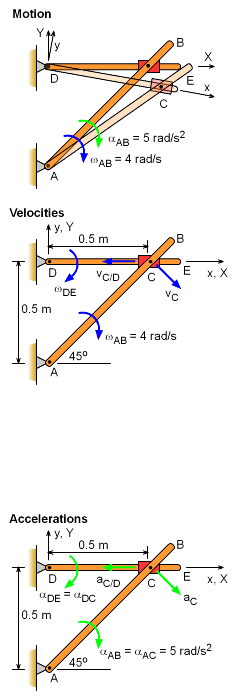Ch 5. Rigid Body General Motion Multimedia Engineering Dynamics Fixed Axis Rotation Plane Motion Velocities Zero Velocity Point Plane Motion Accelerations Multiple Gears Rot. Coord. Velocities Rot. Coord. Acceleration
 Chapter - Particle - 1. General Motion 2. Force & Accel. 3. Energy 4. Momentum - Rigid Body - 5. General Motion 6. Force & Accel. 7. Energy 8. Momentum 9. 3-D Motion 10. Vibrations Appendix Basic Math Units Basic Equations Sections Search eBooks Dynamics Fluids Math Mechanics Statics Thermodynamics Author(s): Kurt Gramoll ©Kurt GramollDYNAMICS - EXAMPLETwo Connected Bars Example Two rods are pinned to a fixed point at one end, and connect to each other at the other end using a slider joint. Point C can freely move along rod DE but is pinned to rod AB at C. Rod AB rotates clockwise with angular velocity ωAB = 4 rad/s and angular acceleration αAB = 5 rad/s2 when θ = 45o. Find the angular acceleration of rod DE at this instant. The collar at C is pin connected to AB and slides over rod DE. SolutionSince the distance between point D and C varies, two frames of reference is useful to solve the problem. Attach the local x-y coordinate to the rotating AE rod, and the global X-Y coordinate to the non-moving background. Velocities: Before accelerations can be found, the velocity of point C, vC/D, and angular velocity of ΩDE (= ΩDC) needs to be determined.      vC = vD + Ω × rC/D + ( vC/D)rel      vC = vD + ΩDC× rC/D + vC/D      vD = 0      ωAB = 4k      rC/D = 0.5 m i      ΩDC = ωDC Since distance from A and C does not change, velocity of C can be determined from bar AB      vC = ωAB × rC/A = -4k × (0.5i + 0.5j)           = 2i - 2j m/s Putting all terms together gives,      2i - 2j = 0 + (-ωDC k) × (0.5i) + vC/D reli      2i - 2j = -0.5ωDC j + vC/D rel i Comparing the i and j terms give      vC/D = 2 m/s   and   ωDC = 4 rad/s Accelerations:      aC = aD + dΩDC/dt × rC/D + ΩDC× (ΩDC × rC/D)               + 2ΩDC × vC/D + aC/D      aC = aD + αDE × rC/D + ωDE× ( ωDE × rC/D)               + 2ωDE × vC/D + aC/D where,      aD = 0      rC/D = 0.5i m      αDC = (-αDCk) × 0.5i = -0.5αDEj m/s2      ωDC × ( ωDC × rC/D) = (- 4)2 (0.5 i) = -8i rad/s      2ωDC × vC/D = 2 (-4k) × 2i = 16j m/s2      aC/D = aC/Di Acceleration of C can be determined from bar AC      aC = αAC × rC/A - ω2 rC/A          = -5k × (0.5i + 0.5j) - (4)2 (0.5i + 0.5j)          = -5.5i - 10.5j m/s2 -5.5i - 10.5j = 0 + (-αDEk)× 0.5i - (- 4)2 (0.5 i)        + 2 (-4k)×2i + (aC/D)i Putting all terms together gives,      -5.5i - 10.5j = 0 - 0.5αDCj - 8i - 16j + aC/Di Comparing the j terms      -10.5 = -16 - 0.5αDE      αDC = -11 rad/s2 αDC = 11k rad/s2 (counter-clockwise)

Practice Homework and Test problems now available in the 'Eng Dynamics' mobile app
Includes over 400 problems with complete detailed solutions.
Available now at the Google Play Store and Apple App Store.